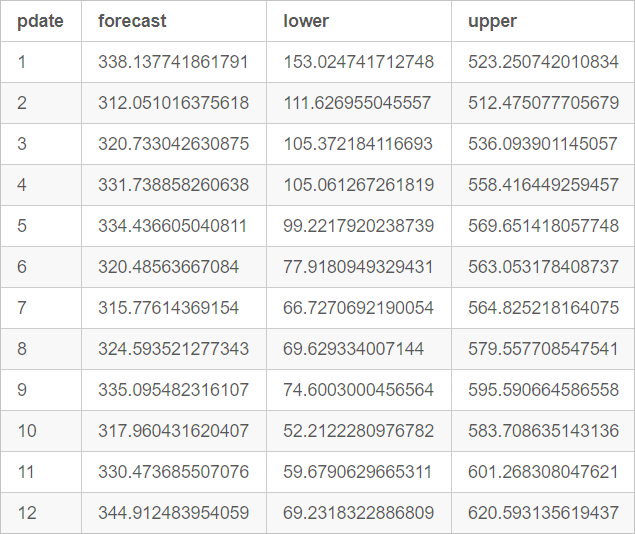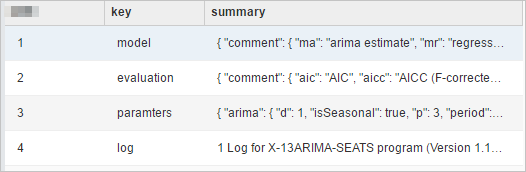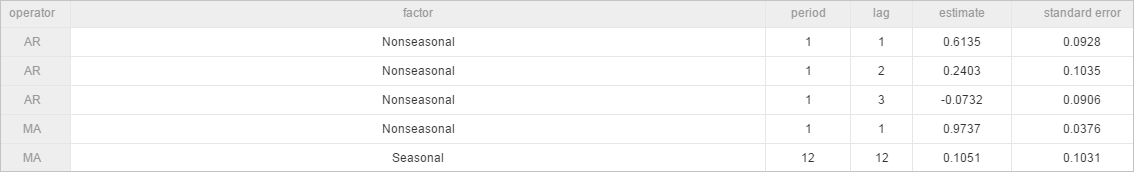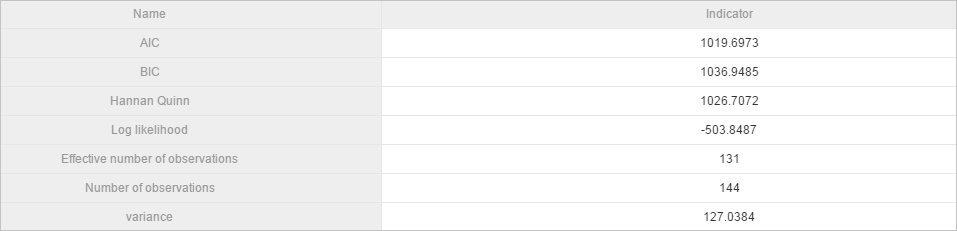X-13-ARIMA is a seasonal adjustment Autoregressive Integrated Moving Average Model (ARIMA) algorithm based on the open source X-13ARIMA-SEATS algorithm.

## Background information

ARIMA was proposed by Box and Jenkins in the early 70s for time series forecasting. ARIMA is also known as Box-Jenkins Model. You can configure the component by using one of the following methods.

## Machine Learning Platform for AI console

The following table describes the parameters of the x13_arima component.
Tab Parameter Description
Fields Setting Time Series Column Required. It is used only to sort numeric values.
Value Column Required.
Stratification Column Optional. You can separate multiple columns with commas (,), such as col0,col1. A time series is created for each group.
Parameters Setting Format The supported format is p,d,q. p, d, and q are non-negative integers in the range of [0, 36].
• p: autoregressive coefficient
• d: difference
• q: moving regression coefficient
Start Date The supported format is year.seasonal. Example: 1986.1.
Series Frequency The value must be a positive integer in the range of [1,12].
Format The supported format is sp,sd,sq. sp, sd, and sq are non-negative integers in the range of [0,36].
• sp: seasonal autoregressive coefficient
• sd: seasonal difference
• sq: seasonal moving regression coefficient
Seasonal Cycle The value must be a number in the range of (0,12]. Default value: 12.
Prediction Entries The value must be a positive integer in the range of (0,120]. Default value: 12.
Prediction Confidence Level The value must be a number in the range of (0,1). Default value: 0.95.
Tuning Cores The number of cores, which is automatically allocated.
Memory Size The memory size of each core. Unit: MB.

## PAI command

``````PAI -name x13_arima
-project algo_public
-DinputTableName=pai_ft_x13_arima_input
-DseqColName=id
-DvalueColName=number
-Dorder=3,1,1
-Dstart=1949.1
-Dfrequency=12
-Dseasonal=0,1,1
-Dperiod=12
-DpredictStep=12
-DoutputPredictTableName=pai_ft_x13_arima_out_predict
-DoutputDetailTableName=pai_ft_x13_arima_out_detail``````
Parameter Required Description Default value
inputTableName Yes The name of the input table. No default value
inputTablePartitions No The names of feature columns selected from the input table for training. Full table
seqColName Yes The series column. This parameter is used only to sort values in the value column. No default value
valueColName Yes The value column. No default value
groupColNames No The grouping columns. You can separate multiple columns with commas (,), such as col0,col1. A time series is created for each group. No default value
order Yes p indicates the autoregressive coefficient. d indicates the difference. q indicates the moving regression coefficient. The value must be a non-negative integer in the range of [0,36]. No default value
start No The start date of a time series. A string in the year.seasonal format, such as 1986.1. For more information, see The time series format. 1.1
frequency No The time series frequency. The value must be a positive integer in the range of (0,12]. For more information, see The time series format. 12
Note 12 indicates 12 months or one year.
seasonal No sp indicates the seasonal autoregressive coefficient. sd indicates the seasonal difference. sq indicates the seasonal moving regression coefficient. The value must be a non-negative integer in the range of [0,36]. No seasonal
period No The seasonal cycle. The value must be a number in the range of (0,100]. frequency
maxiter No The maximum number of iterations. The value must be a positive integer. 1500
tol No The tolerance. The value is of the DOUBLE type. 1e-5
predictStep No The number of prediction entries. The value must be a positive integer in the range of (0,365]. 12
confidenceLevel No The confidence level. The value must be a number in the range of (0,1]. 0.95
outputPredictTableName Yes The output table. No default value
outputDetailTableName Yes The details table. No default value
outputTablePartition No Specifies whether to export data to a partition. Not exporting data to partitions by default
coreNum No The number of cores. The value must be a positive integer. This parameter is used with memSizePerCore. Automatically allocated
memSizePerCore No The memory size of each core. Unit: MB. The value must be a positive integer in the range of [1024,64 × 1024]. Automatically allocated
lifecycle No The lifecycle of the output table. No default value

## The time series format

start and frequency specify the time dimensions ts1 and ts2 for the value column.
• frequency indicates the data frequency within a unit period, which is equal to the frequency of ts2 in each ts1.
• The value of start is in the `n1.n2` format. This indicates that the start date is the n2th ts2 in the n1th ts1.
Unit time ts1 ts2 frequency start
12 months/year Year Month 12 1949.2 indicates the second month of the year 1949.
4 quarters/year Year Quarter 4 1949.2 indicates the second quarter of the year 1949.
7 days/week Week Day 7 1949.2 indicates the second day of the 1949th week.
1 Any time unit 1 1 1949.1 indicates the year 1949, or the 1949th day or hour.
Example: value=[1,2,3,5,6,7,8,9,10,11,12,13,14,15]
• `start=1949.3,frequency=12` indicates that the data is for 12 months and the prediction starts in June of the year 1950.
year Jan Feb Mar Apr May Jun Jul Aug Sep Oct
1949 1 2 3 4 5 6 7 8 9 10
1950 11 12 13 14 15
• `start=1949.3,frequency=4` indicates that the data is for four quarters, and the prediction starts in the second quarter of the year 1953.
year Qtr1 Qtr2 Qtr3 Qtr4
1949 1 2
1950 3 4 5 6
1951 7 8 9 10
1952 11 12 13 14
1953 14 15
• `start=1949.3,frequency=7` indicates that the data is for seven days, and the prediction starts on the fourth day of the 1951st week.
week Sun Mon Tue Wed Thu Fri Sat
1949 1 2 3 4 5
1950 6 7 8 9 10 11 12
1951 13 14 15
• `start=1949.1,frequency=1` indicates that the prediction starts in 1963.00.
cycle p1
1949 1
1950 2
1951 3
1952 4
1953 5
1954 6
1955 7
1956 8
1957 9
1958 10
1959 11
1960 12
1961 13
1962 14
1963 15

## Example

Test data

The AirPassengers dataset is used.
id number
1 112
2 118
3 132
4 129
5 121
... ...
Use the command-line tool tunnel to upload data. Commands:
``````create table pai_ft_x13_arima_input(id bigint,number bigint);
tunnel upload data/airpassengers.csv pai_ft_x13_arima_input -h true;``````
PAI command
``````PAI -name x13_arima
-project algo_public
-DinputTableName=pai_ft_x13_arima_input
-DseqColName=id
-DvalueColName=number
-Dorder=3,1,1
-Dseasonal=0,1,1
-Dstart=1949.1
-Dfrequency=12
-Dperiod=12
-DpredictStep=12
-DoutputPredictTableName=pai_ft_x13_arima_out_predict
-DoutputDetailTableName=pai_ft_x13_arima_out_detail``````
Output
• Output table outputPredictTableName
column name comment
pdate The date of the prediction.
forecast The prediction conclusion.
lower The lower threshold of the prediction results when the confidence level is specified. The default confidence level is 0.95.
upper The upper threshold of the prediction results when the confidence level is specified. The default confidence level is 0.95.
The following figure describes the data.• Output table outputDetailTableName
The following table describes the parameters.
column name comment
key
• model: the model in use.
• evaluation: the evaluation result.
• parameters: training parameters.
• log: training logs.
summary The storage details.
The following figure shows the data.PaiWeb display-model coefficient (key=model)PaiWeb display-evaluation metrics (key=evaluation)## Algorithm scale

• Supported scale
• Row: a maximum of 1,200 data records in a group
• Column: one numeric column
• Resource calculation method
• Default calculation method if groupColNames is not specified:

coreNum = 1

memSizePerCore = 4096

• Default calculation method if groupColNames is specified:

coreNum = floor(Total number of rows/120,000)

memSizePerCore = 4096

## FAQ

Why are prediction results the same?

If an exception occurs during model training, the mean model is called. In this case, all prediction results are the mean of the training data.

Common exceptions include instability after temporal-difference learning, training without convergence, and variance 0. You can view the stderr file of individual nodes in Logview to obtain specific information about exceptions.